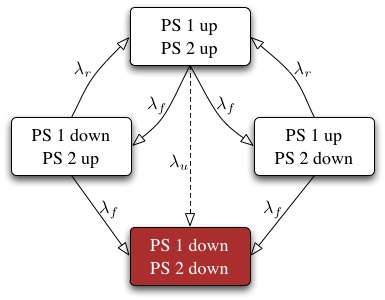PhaseType Package

This package is on CRAN:
PhaseType R Package

So, visit that page to download manually, or from R simply run:
install.packages("PhaseType")

< Back to homepage

This is a package for working with Phase-type (PHT) distributions in the R programming language. The entire of the MCMC portion of the code has been written in optimised C for higher performance and very low memory use, whilst being easy to call from wrapper R functions.

## Definition of a Phase-type Distribution

Consider a continuous-time Markov chain (CTMC) on a finite discrete state space of size (n+1), where one of the states is absorbing. Without loss of generality the generator of the chain can be written in the form:

$\mathbf{T} = \left( \begin{array}{cc} \mathbf{S} & \mathbf{s} \\ \mathbf{0}^\mathrm{T} & 0 \end{array} \right)$

where $$\mathbf{S}$$ is the $$n \times n$$ matrix of transition rates between non-absorbing states; $$\mathbf{s}$$ is an $$n$$ dimensional vector of absorption rates; and $$\mathbf{0}$$ is an $$n$$ dimensional vector of zeros. We take $$\boldsymbol{\pi}$$ as the initial state distribution: an $$n$$ dimensional vector of probabilities $$\left(\sum_i \pi_i=1\right)$$ such that $$\pi_i$$ is the probability of the chain starting in state $$i$$.

Then, we define a Phase-type distribution to be the distribution of the time to absorption of the continuous-time Markov chain with generator $$\mathbf{T}$$, or equivalently as the first passage time to state $$n+1$$. Thus, a Phase-type distribution is a positively supported univariate distribution having distribution and density functions:

$\begin{array}{rcl} F_X(x) &=& 1 - \boldsymbol{\pi}^\mathrm{T} \exp\{x \mathbf{S}\} \mathbf{e}\\ f_X(x) &=& \boldsymbol{\pi}^\mathrm{T} \exp\{x \mathbf{S}\} \mathbf{s} \end{array} \qquad \mbox{for } x \in [0,\infty)$

where $$\mathbf{e}$$ is an $$n$$ dimensional vector of $$1$$'s; $$x$$ is the time to absorption (or equivalently first-passage time to state $$n+1$$); and $$\exp\{x \mathbf{S}\}$$ is the matrix exponential. We denote that a random variable $$X$$ is Phase-type distributed with parameters $$\boldsymbol{\pi}$$ and $$\mathbf{T}$$ by $$X \sim \mathrm{PHT}(\boldsymbol{\pi},\mathbf{T})$$.

Note that $$\displaystyle \sum_{j=1}^n S_{ij} = -s_i \ \forall\,i$$, so often a Phase-type is defined merely by providing $$\mathbf{S}$$, $$\mathbf{T}$$ then being implicitly known.

## Example

Phase-types are highly flexible, not least because they are dense in the function space of all positively supported probability distributions (Asmussen 2000). Consequently, given a sufficiently large generator $$\mathbf{T}$$, any distribution which is positive only on $$[0,\infty)$$ can be arbitrarily closely approximated by a Phase-type. This makes them an interesting alternative to mixtures of Gaussians for approximating positively supported distributions. Bladt et al. (2003) provide a Bayesian inferential procedure based on MCMC to perform distribution fitting with Phase-types.

Alternatively, since the latent process involved in Phase-types is a continuous-time Markov chain, they also provide a good modelling tool for certain stochastic processes. For example, consider a repairable redundant system of two electronic components, PS1 and PS2. The stochastic process leading to ultimate system failure can be visualised graphically:This model is represented by the continuous-time Markov chain generator (ignoring $$\lambda_u$$):

$\mathbf{T} = \left(\begin{array}{cccc} -2\lambda_\mathrm{f} & \lambda_\mathrm{f} & \lambda_\mathrm{f} & 0 \\ \lambda_\mathrm{r} & -\lambda_\mathrm{r}-\lambda_\mathrm{f} & 0 & \lambda_\mathrm{f} \\ \lambda_\mathrm{r} & 0 & -\lambda_\mathrm{r}-\lambda_\mathrm{f} & \lambda_\mathrm{f} \\ 0 & 0 & 0 & 0 \end{array}\right)$

If we define $$\boldsymbol{\pi} = (1,0,0)^\mathrm{T}$$, then the distribution of the time to failure from full operation is Phase-type with initial distribution $$\boldsymbol{\pi}$$ and generator $$\mathbf{T}$$. Bayesian inference in this second scenario is addressed by Aslett and Wilson (2011).

## The R Package

At present, the R package contains just two publicly visible functions: one to perform descriptive or statistical model inference as per Bladt et al. (2003); and another to perform mechanistic or stochastic model inference as per Aslett and Wilson (2011).

Code which simulates data from the above example stochastic model — for $$\lambda_f=1.8$$ and $$\lambda_r=9.5$$ — and then performs the two kinds of inference is as follows: (NB the package actuar contains functions for generating random variates of Phase-type: a future version of the PhaseType package will provide high-performance functions for this directly)

library(actuar)
# Define the S matrix (columnwise)
S <- matrix(c(-3.6, 9.5, 9.5, 1.8, -11.3, 0, 1.8, 0, -11.3), 3)

# Define starting state distribution
pi <- c(1, 0, 0)

# Generate 50 random absorption times from the Phase-type with
# subgenerator S and starting distribution pi, which we will
# try to infer next
x <- rphtype(50, pi, S)

library(PhaseType)
# FIRST: descriptive model fit (Bladt et al. 2003)
# Prior on starting state
dirpi <- c(1, 0, 0)

# Gamma prior: shape hyperparameters (one per matrix element,
# columnwise)
nu <- c(24, 24, 1, 180, 1, 24, 180, 1, 24)

# Gamma prior: reciprocal scale hyperparameters (one per matrix
# row)
zeta <- c(16, 16, 16)

# Define dimension of model to fit
n <- 3

# Perform 10000 MCMC iterations (fix inner Metropolis-Hastings to
# one iteration since starts in stationarity here).
res1 <- phtMCMC(x, n, dirpi, nu, zeta, 10000, mhit=1)
print(res1)
plot(res1)

# SECOND: mechanistic model fit (Aslett and Wilson 2011)
# Prior on starting state
dirpi <- c(1, 0, 0)

# Define the structure of the Phase-type generator
T <- matrix(c(0,"R","R",0,"F",0,0,0,"F",0,0,0,0,"F","F",0), 4)

# Gamma prior: shape hyperparameters (one per model parameter)
nu <- list("R"=180, "F"=24)

# Gamma prior: reciprocal scale hyperparameters (one per model
# parameter)
zeta <- c("R"=16,"F"=16)

# Perform 10000 MCMC iterations.
res2 <- phtMCMC2(x, T, dirpi, nu, zeta, 10000)
print(res2)
plot(res2)


## References

Aslett, L. J. M. & Wilson, S. P. (2011), Markov chain Monte Carlo for inference on Phase-type models, Technical report, Trinity College Dublin (pending journal submission).

Asmussen, S. (2000), ‘Matrix-analytic models and their analysis’, Scandinavian Journal of Statistics 27(2), 193–226.

Bladt, M., Gonzalez, A. & Lauritzen, S. L. (2003), ‘The estimation of phase-type related functionals using Markov chain Monte Carlo methods’, Scandinavian Journal of Statistics 2003(4), 280–300.